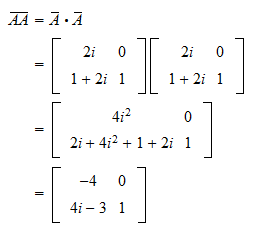StatLect

# Complex vectors and matrices

Up to this point, we have progressed in our study of linear algebra without ever specifying whether the entries of our vectors and matrices are real or complex numbers. Although the examples and exercises presented thus far concern real matrices (i.e., matrices having real entries), all the definitions, propositions and results found in previous lectures are applicable without modification to complex matrices (i.e., matrices whose entries are complex numbers). In fact, if you revise those lectures, you will realize that nowhere, and especially in no proof, it is necessary to assume that a matrix or vector be real. The only caveat is that when we deal with complex matrices, we also need to use complex scalars when taking linear combinations.

In this lecture, we are going to revise some elementary facts about complex numbers. We then show some basic properties of complex matrices and provide some useful definitions.## Complex numbers

A complex numberis a number that can be written aswhereandare real numbers, called the real and imaginary part of the complex number respectively, andis called imaginary unit.

Hence, when we manipulate complex numbers, the key "trick" we exploit over and over again isImaginary numbers allow us to find solutions to equations that have no real solutions. For example, the equationhas no real solution, but it has two imaginary solutionsReal numbers are complex numbers that have zero imaginary part. The latter is often omitted, that is, instead of writingwe simply write.

### Complex conjugate

An important concept is that of complex conjugate. Given a complex numberits conjugate, denoted by, isAs a consequence, double conjugation leaves numbers unchanged:### Algebra of complex numbers

The algebra of complex numbers is similar to the algebra of real numbers. Given two complex numberswe have the following rules:

1. Addition: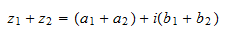2. Subtraction: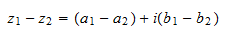3. Multiplication: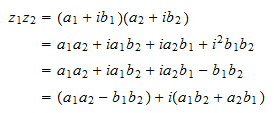4. Division: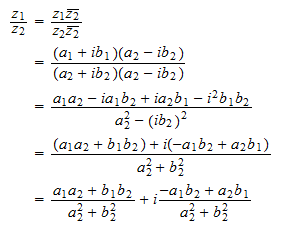### Distributive properties of conjugation

Note that conjugation is distributive under addition: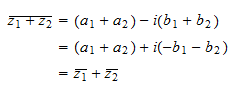and under multiplication: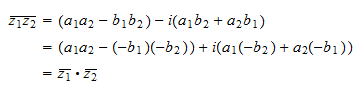### Modulus of a complex number

The modulus (or absolute value) of a complex numberis defined aswhere we consider only the positive root.

Clearly, the modulus is always a real number.

## Complex matrices

Complex matrices (and vectors) are matrices whose entries are complex numbers.

### Complex conjugation of matrices

Given amatrix, its complex conjugateis the matrix such thatthat is, the-th entry ofis equal to the complex conjugate of the-th entry of, for anyand.

Example Define the matrixThen its complex conjugate is### Distributive properties of conjugation

The distributive properties that hold for the conjugation of complex numbers hold also for the conjugation of matrices.

Proposition Ifandare twomatrices, thenProof

We have thatfor anyand, by the distributive property of the conjugation of complex numbers under addition.

Proposition Ifismatrix andis amatrix, thenProof

We have thatfor anyand, by the distributive property of the conjugation of complex numbers under addition and multiplication.

Proposition Ifis amatrix andis a scalar, thenProof

We havefor anyand, by the definition of multiplication of a matrix by a scalar and by the distributive property of the conjugation of complex numbers under multiplication.

### Conjugate of a real matrix

A trivial but useful property is that taking the conjugate of a matrix that has only real entries does not change the matrix. In other words, ifhas only real entries, thenThis is a consequence of the fact that a real number can be seen as a complex number with zero imaginary part. But all that conjugation does is to change the sign of the imaginary part of a complex number. Therefore, a real number is equal to its conjugate.

## Solved exercises

Below you can find some exercises with explained solutions.

### Exercise 1

Define two vectors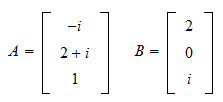Compute the following modulus:Solution

The product ofandis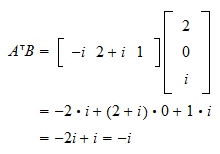and its modulus is### Exercise 2

DefineandComputeSolution

First of all, we can substituteinto:The complex conjugate ofisThe product we need to compute is